# S-integer

(diff) ← Older revision | Latest revision (diff) | Newer revision → (diff)

As a simple example, let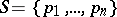be a finite set of rational prime numbers. The rational integers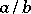,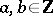, relatively prime (cf. also Mutually-prime numbers), such that the set of prime divisors of(possibly empty) is contained inare the so-called-integers (corresponding to the specific set). Clearly, this is a subring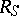of. Letdenote the group of units of, i.e. the group of multiplicatively invertible elements of(the-units). Clearly, these areand the rational numbersin the prime decomposition of which only prime numbers from the setappear.

These notions can be defined in a more sophisticated way, the advantage of which is that it can be generalized to the more general case of a number field. For this the notion of absolute value on a number field is needed. Unfortunately, there is no general agreement on the definition of this notion. Below, this "absolute value" is taken in the sense of a metric as in [a1], Chap. 1, Sect. 4; Chap. 4, Sect. 4; equivalently, an absolute value is a function, whereis a fixed, conveniently chosen positive real number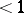andis a valuation, as defined and used in [a2], Chap. 1, § 2; Chap. 3 § 1, (cf. also Valuation, which gives a slightly different definition).

In the special case above, every rational prime numbergives rise to a-adic absolute value and all possible absolute values ofare (up to topological equivalence) the-adic ones (non-Archimedean), denoted by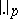, and the usual absolute value (Archimedean), denoted by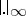. Letdenote the set of absolute values (more precisely, the set of equivalence classes of absolute values (i.e. places) of; cf. also Place of a field). Thus, every element of this set is of the form, whereis either a rational prime number or the symbol. One now modifies the definition of the setabove as the subset of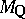containing the absolute values (i.e. places), where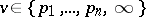. Then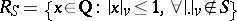and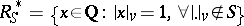.

Consider now the more general situation, where a number fieldis taken in place ofand its ring of integers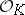is taken in place of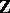. Letbe the set of absolute values of(more precisely, the set of equivalence classes of absolute values, i.e. places, of). These are divided into two categories, namely, the non-Archimedean ones, which are in one-to-one correspondence with the prime ideals (or, what is essentially the same, with the prime divisors) ofand the Archimedean ones, which are in one-to-one correspondence with the isomorphic embeddings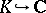(complex-conjugate embeddings giving rise to the same absolute value). As before, letbe a finite subset of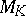containing all Archimedean valuations of. Then, the setof-integers and the setof-units are defined exactly as in the case of rational numbers (see the definitions above), where nowis replaced by.

Many interesting problems concerning the solution of Diophantine equations are reduced to questions about-integers of "particularly simple form" (e.g. linear forms in two unknown parameters), which are-units, and then results are obtained by applying a variety of relevant results on-integers and-units.

How to Cite This Entry:
S-integer. Encyclopedia of Mathematics. URL: http://encyclopediaofmath.org/index.php?title=S-integer&oldid=15023
This article was adapted from an original article by N. Tzanakis (originator), which appeared in Encyclopedia of Mathematics - ISBN 1402006098. See original article